# Explanation of Acid – Base Problems

## TITRATION AND pH PROBLEMS

THE FOLLOWING SALTS IN WATER SOLUTION WILL HAVE A pH OF 7, -7(less than 7), +7(more
than 7), OR INDETERMINATE (I). FOR EACH PREDICT THE pH OF THE SOLUTION.

1. Na2CO3
is a basic salt because NaOH is a strong base and H2CO3 is a weak acid. pH is above 7.

2. FeCl3 is an acid salt because
Fe(OH)3 is weak base and HCl is a strong acid. pH is less than 7.

3. KNO3 is a neutral
salt. Both HNO3 and KOH are strong. pH = 7.

4. NH4C2H3O2
is indeterminate. Both NH4OH
and HC2H3O2
are weak. pH is actually only a very little above 7.

5. ZnSO4 is an acid salt. The first ionization of
H2SO4
is strong and Zn(OH)2 is
weak. pH is less than 7.

6. Ba(NO3)2
is neutral because both Ba(OH)2
and HNO3 are strong. pH = 7.

7. RbF is an alkaline salt because RbOH is strong and HF is weak. pH is over 7.

8. CaBr2 is neutral because
both Ca(OH)2 and HBr are
strong. pH = 7.

9. What is the pH of a 0.0115 M HCl solution?

The only two materials in the solution are water and HCl. The water alone
would provide 1 E-7 Molar hydrogen ion. The HCl is a strong and soluble acid,
so all of the acid is in the form of ions, providing 0.0115 Molar hydrogen ion.
There is no need to consider the hydrogen ion from water as it is much less than
five percent of the total hydrogen ion concentration.
The concentration of hydrogen ion is adequately estimated at 0.0115 M.
This is only a pH box
We suggest rounding pH values to one decimal place. Few pH meters
are that accurate.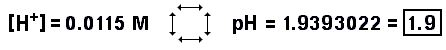10. Find the pH of 0.0815 M NaOH solution.

The only two materials in the solution are water and NaOH. The water alone
would provide 1 E-7 Molar hydroxide ion. The NaOH is a strong and soluble base,
so all of the base is in the form of ions, providing 0.0815 Molar hydroxide ion.
The hydroxide ion is most important in this problem. The hydroxide ion from the
dissociation of water is much less than five percent of the hydroxide ion from
sodium hydroxide, so the concentration
of hydroxide ion is 0.0815 M. This is only a pH box
problem. The answer has been rounded to one decimal place.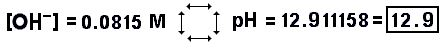11. Find the pH of 0.00372 M Ba(OH)2 solution.

Ba(OH)2 is a strong base,
that is, up to its solubility limits the compound will be completely ionized
in water solution. [(OH)]
is twice the [Ba(OH)2]
because for each mol of Ba(OH)2
there are two mols of hydroxide ion. The ionization of water, the only
other possible source of hydroxide ions, produces nowhere near
five percent of the hydroxide ion available from
Ba(OH)2, and so:

[(OH)] = 2
[Ba(OH)2] = 2 (0.00372 M) =
0.00744 M
Now that we know the [(OH)],
this is only a pH box problem. The answer is in
pH, so it is rounded to one decimal place.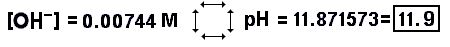12. Find the pH of 0.12 M HC2H3O2

The only two things in this solution are water and acetic acid, a weak acid. The

kA of acetic acid is 1.74 E-5.
Water and acetic acid could contribute hydrogen ion to this mixture. If water
were the only possible contributor, it would give 1 E-7 M hydrogen ion. If the
acetic acid were the only contributor, we could calculate it from the equilibrium
expression. Since the hydrogen ion from the acetic acid would be exactly the
same concentration as the acetate ion, [H+]
= [(C2H3O2)].
And we can simplify by substituting [H+]2
for [H+]
[(C2H3O2)].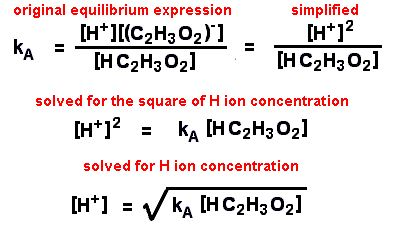Now we can substitute our numbers for the
kA
and [HC2H3O2]
to find that [H+] =
the square root of (0.12 x 1.74 E-5). The [H+]
due to acetic acid is 1.4450 E-3 M.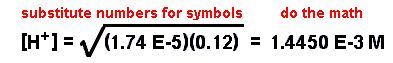The [H+] from
the acetic acid is less than five percent> of the
[acetic acid], so there is no need to get a more accurate answer by subtracting
the [H+] from the
[acetic acid]. After finding the [H+],
we calculate the pH by using the pH box. The pH
is 2.840134, rounded to 2.8.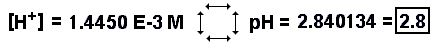13. Calculate the pH of 0.0000135 M H3BO3

Oh, no. We have a complication. H2CO3
has such a small kA (5.37
E-10 for the first ionization) and the acid is in such small concentrations
that the [H+] from
the acid will be not much more than the [H+]
from the ionization of water. The other possible sources of
[H+] are the second
and third ionizations of the boric acid, but they both have
kA‘s that are far from the
first ionization, so they should not be of any significance.

What you would find, if you assume the ionization of water to be negligible,
is a silly answer. You try it. Use the standard square root of (
kA times
[H3BO3]).
You get a pH OVER seven. (pH = 7.1) Ridiculous. Boric acid is an acid
and should result in a pH of less than seven.

There are several ways to do this. One way is by successive approximations.
First use the ionization constant formula to calculate the
[H+]
from the acid, then use that [H+]
to find the [OH].
Since the [H+] from
water equals the [OH],
you can add that number back to the [H+]
from the acid to get a first round total [H+].
Use the first round total [H+]
in the acid dissociation equation to find the concentration of acetate ion and
that would be equal to the second round estimation of the [H+]
from acid. Keep going around that circle until you get three stable
significant digits for the total [H+].

Another way is by substitutions into known equations. Your equations are
the formula for the kw,
the formula for the [H+]
of a weak acid, the equality of the [H+]
from water with the [OH],
and the statement that the total [H+]
equals the [H+] from
water plus the [H+]
from the ionization of the acid.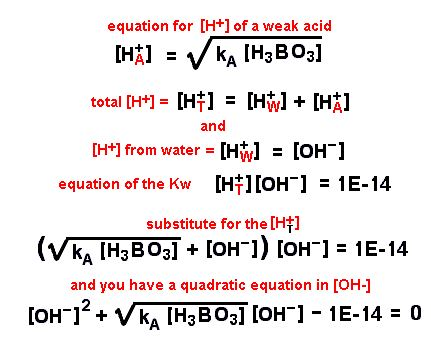Or you could substitute in for the [OH]
ion and solve for the [H+].

Drop the numbers in and do the math. It is messy and tedious and disgusting
to have to use the quadratic equation, but it will give you and answer of
[OH] = 6.6115 E-8
which results in a pH of 6.8203, rounded to 6.8, a likely answer.

14. pH of 0.255 M NH4OH

The kB
of NH4OH is 1.78 E-5.

The ionization equilibrium expression for NH4OH
or any other weak base is similar to the ionization equilibrium expression for a weak
acid, but this time the equation will be solved for the hydroxide ion concentration.

The hydroxide ion is in excess. The two sources of hydroxide ion
are the [H+] and
water, but the contribution from the water is negligible.

The other assumption, that the amount of dissociated ion is negligible, is
also valid because the concentration of base is so large. (Try it if you
don’t believe it.)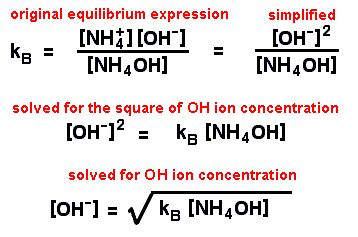Now substitute the numbers and do the math.15. 0.578 M H3PO4

In a solution that has only water and phosphoric acid there are four possible
sources of hydrogen ion, the water and the three ionizations of the phosphoric
acid. Each ionization has a different
kA.

 H3PO4 ===> (H2PO4)– + H+ first ionization kA = 6.92 E-3 (– ===> (HPO4)2– + H+ second ionizaton kA = 6.17 E-8 (HPO4)2– ===> (PO4)3– + H+ third ionization kA = 2.09 E-12

It is clear that the first ionization of phosphoric acid at this
concentration is much greater than the third
ionization of phosphoric acid, but the big questions are whether we
need to consider the second ionization of phosphoric acid or the ionization of water.

The hydrogen ion concentration from the first ionization can be calculated
by the usual square root of ([acid]kA)
to get an initial estimate.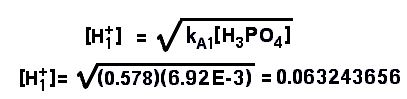From the initial estimate, the [H+]
from the first ionization (0.063243656 M) will be more than 5% of the
acid concentration (0.578 M). We will have to use the more accurate form of the equation that includes the
decrease in unionized acid concentration by the ionized forms.

Will we have to also account for the second ionization? The number we found
as first estimate for the [H+]
can also serve as estimate of the [H2PO4)],
the anion in the first ionization. But the anion of the first ionization is
the original acid in the second ionization and the estimate of the
[H+] can also be
used in the second ionization equation. If we solve for the anion of the
second ionization equation, we would get an estimate of the hydrogen ion that
the second equation would produce.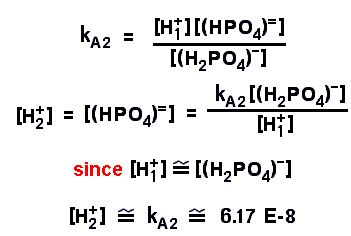This is an interesting result. Notice the [H+]
from the second ionization is very close to the second kA
when there is nothing but water and phosphoric acid in the solution. What would
happen at other concentrations of phosphoric acid? At other pH’s whith the same
initial concentration of phosphoric acid?

The concentration estimate of the hydrogen ion from the second ionization
is far below 5% of the hydrogen ion from the first ionization, so the second
ionization does not need to be considered. But we do need to consider the
decrease in acid concentration from ionization of the first reaction, so we
use the quadratic form of the equation substituted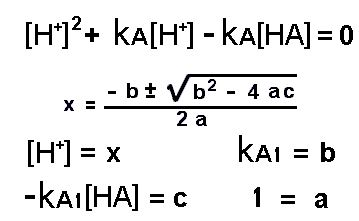When you substitute the numbers, the math looks like: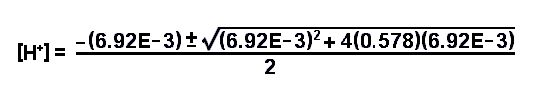As you can see when you do the math, the [H+]
is 0.059878231, more than 5% different from the original estimate. Now we can
confidently change that [H+]
to the pH by the pH box.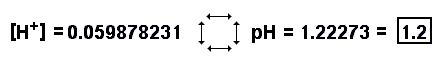If you are really sharp, you noticed that the original estimate using
the simplified method of calculating the [H+]
produces the number 0.063243656, and that the pH of that [H+]
is the SAME as the number from the more complex calculation when rounded to
one decimal point.

16. What is the pH of 0.16 M HCl and 0.072 M phosphoric acid?

There are five sources of hydrogen ion in this solution. Count them. Water,
HCl, and the three ionizations of the phosphoric acid. Likely the water and the
lesser two of the three ionizations of phosphoric acid will not significantly
contribute to the hydrogen ion concentration.

Since the HCL is a strong acid, it contributes 0.16 Molar hydrogen ion to
the solution, but the phosphoric acid is a weak acid and the [H+]
from it can not calculated by the square root of (kA
[H3PO4])
because the [H+] is
not equal to the [H2PO4].
We must go back to the ionization equilibrium equation for the first ionization
of phosphoric acid.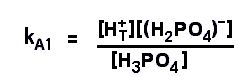We can look up the kA.
The [H+] is the same
as the [HCl], and the concentration of phosphoric acid is given. The
[H2PO4]
will be equal to the concentration of hydrogen ion contributed by the first
ionization of the phosphoric acid.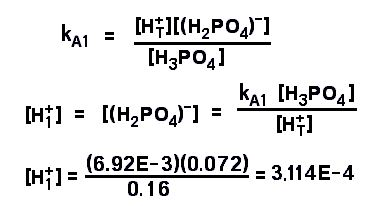So the pH depends only on the [HCl].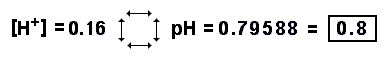17. Find the pH of 1.25 M acetic acid and 0.75 M potassium acetate.

Acetic acid kA
= 1.74 E-5       pKA
= 4.76.

This is a genuine buffer problem. Added to the water are a weak acid and
a salt containing the anion of the acid.

There are two good ways to work buffer problems, with the Henderson- Hasselbach
equation or with the ionization equilibrium expression of the weak acid or base.
I personally have a mental block against the H-H equation because I can never
remember whether it uses a positive or negative log and which concentration
goes on top. You can use it if you wish. Particularly if you need to calculate
buffers often, you should engrave it upon your gray matter. If you really need it
and can’t remember it, you can derive it from the ionization equilibrium
expression.

There are three cautions you need to observe with either equation: (1) Make
sure you are using the correct concentration for each variable, (2) check
to see if the numbers you propose to use are going to be within the 5% rule
for simplification, and (3) estimate the answer from what you know and make sure

Before actually doing the problem, estimate the answer from your own reasoning.
In this case, the pkA of acetic
acid is 4.76. The rule is that an equimolar buffer has a pH equal to the
pkA and in this problem
there is less potassium acetate than acetic acid, so the pH must be lower
(more acid) than the pkA
within a pH unit or so. If the acetic acid were the only solute, the pH estimate
would be the square root of (acid concentration times kA).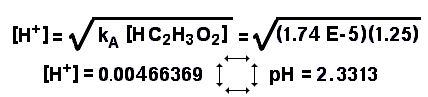The answer should be somewhere between pH of 2.3 and 4.8

The majority of the acetate ion will be from the potassium acetate. Is it
right that the total acetate ion concentration will be equal to the concentration
of the potassium acetate? Or will the acetate ion concentration from the
ionization of the acetic acid contribute more than 5%? The potassium acetate
concentration is 0.75 M. The acetate ion concentration from acetic acid would
be 0.00466 M, less than 5% of 0.75 M even without the common ion effect.
We can safely use 0.75 as the concentration of acetate ion.

Will the concentration of unionized acid be a problem? The measured concentration
is 1.25 M and the ionized amount is 0.00466 M, far less than 5% of 1.25 M.

As threatened, we can use the ionization equilibrium expression of acetic
acid for the main equation for this problem, substituting for the
kA, substituting the
concentration of potassium acetate for the concentration of acetate,
substituting the concentration of acetic acid, and solving for the hydrogen
ion concentration to get the pH.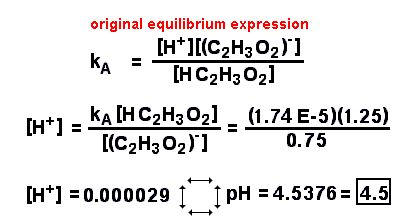The answer of pH = 4.5 is a reasonable one by our estimation because it is
more acid than the pkA of 4.76.

It is a little easier to do this problem by the
Henderson- Hasselbach equation, if you are sure you know it. You must still
make sure you are substituting correctly and that your assumptions for
simplification are valid (within 5%). The H-H equation is not much good for
solutions in which either the acid or ion concentrations are more than ten
times one another or in which the concentration of either material is less
than one hundred times the kA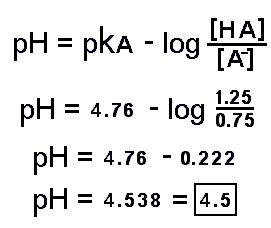18. 0.788 M lactic acid and 1.27 M calcium lactate.

Lactic acid kA
= 8.32 E-4       pKA
= 3.08.

Here is another acid – conjugate base buffer pair. This time there is more
conjugate anion than acid concentration, so we expect the pH to be somewhat
higher (more alkali) than the pKA.
As in the previous problem, there seems to be no complication with either
of the components being of too small a concentration or the concentrations being
too close to the KA,
so there should be no need for a quadratic equation.

The concentration of calcium lactate needs to be doubled (!) to represent
the lactate ion concentration because the calcium is divalent and has two
lactate ions per formula of calcium lactate. The concenration of acid is more
than 100 times the KA,
so the concentration of acid is close enough to the concentration of unionized
species.

By the ionization equilibrium equation: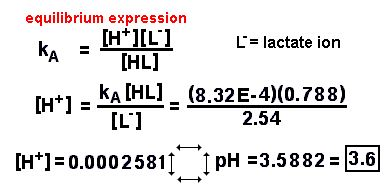Or by the H-H equation, you get the same answer.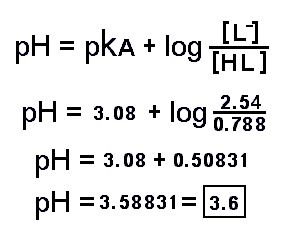19. 0.590 M ammonium hydroxide and 1.57 M ammonium chloride.

ammonium hydroxide kB
= 1.78 E-5       pKB
= 4.75.

Here we have a weak base and its conjugate cation. We can use the ionization
equilibrium expression, but it is different from the acid ionization expression.
The ammonium hydroxide ionizes into hydroxide ion and ammonium ion, so it
would be best to find the concentration of the hydroxide ion.

The ionization
equilibrium expression must have the kB rather than a
kA, or the Henderson-
Hasselbach equation has to have all its components adapted to alkali, but it
is completely analagous to the acid calculation. In either way of doing the
problem, you will have to change the answer to the pH by the pH box.

Will we be able to use our standard shortcuts? The concentration of base is
more than 100 times the kA,
so the measured amount of ammonium hydroxide in solution is a good enough
number for the concentration of unionized species. The concentration of weak
base and conjugate ion will be within 1:10 of each other, so the amount of
conjugate ion can be adequately estimated by the concentration of ammonium
chloride. There is high enough concentration of the base so that the ionization
of water does not significantly change the hydroxide concentration.Or by the H-H equation, you get the same answer.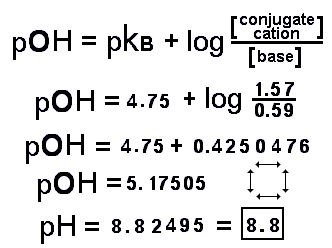Does the answer make sense? The combination is a base buffer and the pH is
slightly base. There is almost three times the concentration of ammonium chloride
than ammonium hydroxide, so the pH of the mixture is more acidic than it would
be if the buffer had been equimolar. (pH = 9.25)

20. Explain how to make 5 L of 0.15 M acetic acid-sodium acetate buffer at
pH 5.00 if you have 1.00 Molar acetic acid and crystaline sodium acetate.

Here is a problem you may have to actually use one day. In biochemistry some
enzymes need to be at a particular pH to work at maximum. You would choose a
weak acid with a pkA close
to the pH you need. (The pkA
of acetic acid is 4.76.) The osmolarity (the total molar amount of dissolved
materials) may be specified. (It is here. The total of acetic acid and sodium
acetate should be at 0.15 Molar.)

It is most convenient to use the Henderson – Hasselbach equation for this,
as it has a term that can be the ratio of the two materials. The form of the
H-H equation does not matter, but the concentration of the conjugate ion will
have to be greater than the concentration of the acid because the pH is
greater than the pkA of
the weak acid.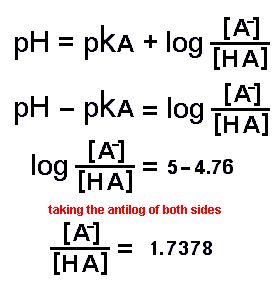What we get from the H-H equation is the ratio of the two constituents. We can
use that ratio as one of the equations in a two – equation – two – unknown
setup to substitute one into the other and calculate the concentration of
acetic acid, [HA], and the concentration of sodium acetate,
[A_].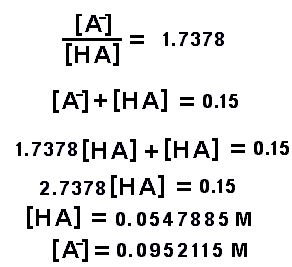But we still have not answered the question, "Explain how to make 5 L of
pH 5, 0.15 M acetic acid-sodium acetate buffer." We have a 1.00 Molar solution
of acetic acid and crystals of (solid) sodium acetate. The way we have to
measure the acetic acid is by measuring the volume of the more concentrated
solution. The way to measure the sodium acetate is to weigh it. We would need
(54.7885 x 5 = 273.9425) ml of acetic acid and (82.04 x 0.0952115 x 5 =
39.055757) grams of sodium acetate.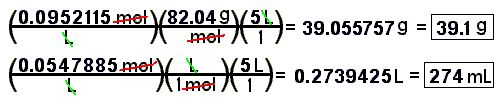The real answer is that you need to weigh 39.1 g of sodium acetate,
measure 274 ml of the 1.00 Molar acetic acid and put them into a 5 liter
volumetric flask with enough water to dissolve the sodium acetate. Then fill
the volumetric flast to the line with distilled water and mix the solution.

FOLLOWING ARE TITRATION PROBLEMS. ASSUME THAT THE pH INDICATOR WILL BE THE RIGHT ONE TO
BALANCE THE AMOUNT OF ACID AND BASE. SOME OF THE TITRATIONS ARE NOT ACID –  BASE
TITRATIONS. AGAIN ASSUME THERE IS AN INDICATOR THAT WILL TELL WHEN MOLAR AMOUNTS ARE
MATCHED.

21. 23.45 mL of 0.275 M sodium hydroxide was used to titrate against mL of acetic acid.
What was the concentration in M of acetic acid?

22. 17.05 mL of 0.247 M barium hydroxide was used to titrate against 10 mL of nitric
acid. What was the concentration in M of nitric acid?

23. 35.79 mL of 0.275 M sodium hydroxide was used to titrate against 15 mL of sulfuric
acid. What was the concentration in M of sulfuric acid?

24. 24.92 mL of 0.00199 M silver nitrate was used to titrate against 5 mL of sodium
chloride solution. What was the concentration of NaCl?